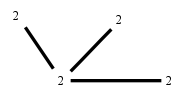# The Markov Bases Database

## BM4r2-2_bin

The binary graphical model/graph model of the tree G13.
loopless Binary Matroid Model of rank 2
2nd of 3 on 4 binary variables

It is a hierarchical model of 4 variables. The dimension of the model is 7.The cardinality of the statespace is 16.

### Properties of the Markov basis

 Markov degree 2 18

 degree 2 18

The model has the following properties:

• All variables are binary.
• It is a graph model and a graphical model.
• The semigroup is normal.### FILES

 Markov basis: sufficient statistics matrix: BM4r2-2_bin.mar (888 b) BM4r2-2_bin.mat (402 b) BM4r2-2_bin.mod (33 b) BM4r2-2_bin.tar.gz (3.94 kb)

Wrong or missing information? Write us an email.

page last update on November 15 2020. ->Contact